## Introduction

We know that there are different number systems - natural numbers, whole numbers, and integers. Watch this video to understand why we need a new number system.

So, from the video you know why we need a new number system - rational numbers.

A rational number is a number that can be written in the form p q where ‘p’ and ‘q’ are integers, and q ≠ 0.

Some examples of rational numbers are: -12, 0, 3 5, -7 9, 120.

#### Concepts

The chapter ‘Rational Numbers’ covers the following concepts:

#### Operations with Rational Numbers

Any natural number, whole number, or integer can be expressed in the form of p q where ‘p’ and ‘q’ are integers, and q ≠ 0. Any natural number, whole number, or integer can also be written with denominator 1.

0 is also a rational number as it can be written as a number with denominator 1.

The table below is a checklist to find out whether a number is a rational number or not.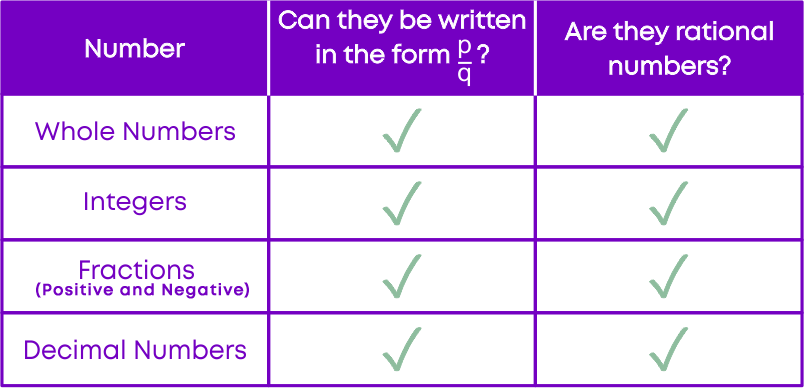When you come across expressions with multiple operations, follow these steps to solve:

• Perform operations in brackets first.
• Then, perform division if any.
• Then, take up multiplication.
• Addition and subtraction have the same preference.
• Follow the rules of signs.

#### Closure Property of Rational Numbers

If ‘a’ and ‘b’ are rational numbers, then the sum a + b is also a rational number.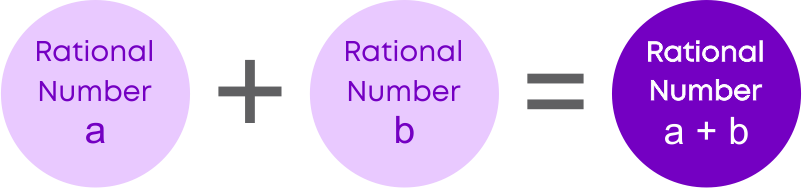This means that rational numbers are closed under addition.

If ‘a’ and ‘b’ are rational numbers, then the product a × b is also a rational number.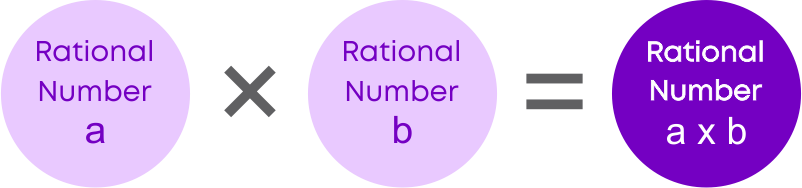This means that rational numbers are closed under multiplication.

In general, if ‘a’ and ‘b’ are rational numbers, the quotient a ÷ b will not always be a rational number.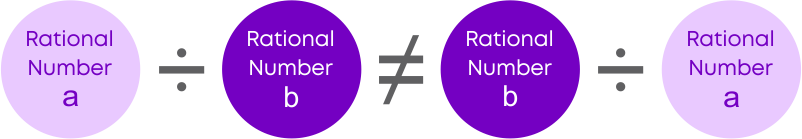So, we can say that rational numbers are not closed under division.

Closure property: when we take any two numbers of the same set of whole numbers/natural numbers/integers and perform a mathematical operation with them, and if the result is also a number of the same set, then the operation is said to be closed for that set.

#### Commutative Property of Rational Numbers

Rational numbers are commutative under addition. If ‘a’ and ‘b’ are rational numbers,

then a + b = b + a. Example: -1 3 + 3 4 = 3 4 + -1 3 = 5 12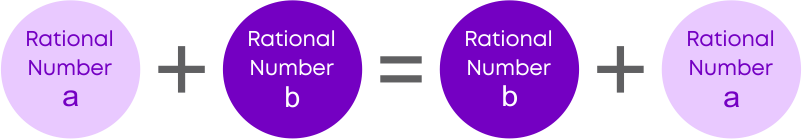Rational numbers are not commutative under subtraction. For any two rational numbers, ‘a’ and ‘b’, a – b ≠ b – a. Example: -1 3 - 3 4 = -13 12 and 3 4 - -1 3 = 13 12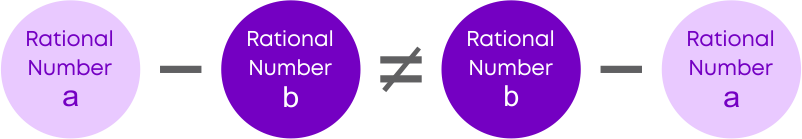Rational numbers are commutative under multiplication. For any two rational numbers, ‘a’ and ‘b’, a × b = b × a. Example: -1 3 × 3 4 = 3 4 × -1 3 = -1 4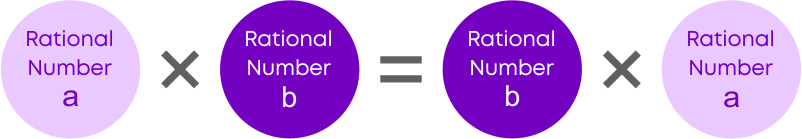Rational numbers are not commutative under division. In general, for two rational numbers, ‘a’ and ‘b’, a ÷ b ≠ b ÷ a. Example: -1 3 ÷ 3 4 = -4 9 and 3 4 ÷ ( -1 3 ) = -9 4Commutative property: the sum or product of the numbers remains the same even if we change the order in which we are adding or multiplying.

#### Associative Property of Rational Numbers

We know that when we add or multiply 3 whole numbers or integers, we can group the numbers to do our addition or multiplication.

• We can also change the order of grouping.
• The order of grouping does not change the answer.

Hence, numbers are associative under addition and multiplication. The same applies to rational numbers.

If ‘a’, ‘b’ and ‘c’ are three rational numbers, then

(a + b) + c = a + (b + c)

• Rational numbers are associative under addition.
• We can group rational numbers to add easily.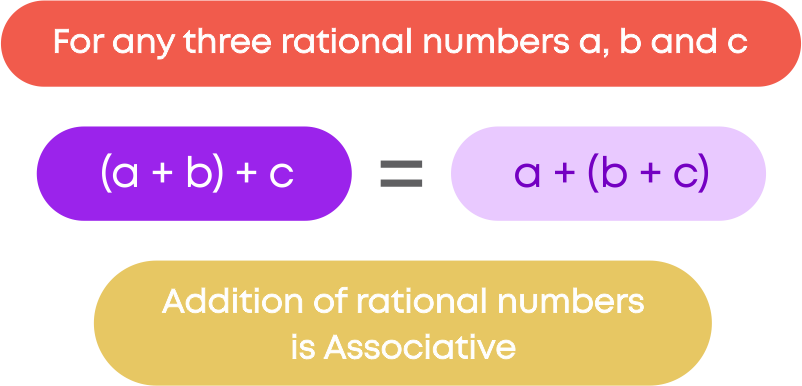• In subtraction, if we change the grouping of the numbers, then the answer will change.
• Subtraction is not associative for rational numbers.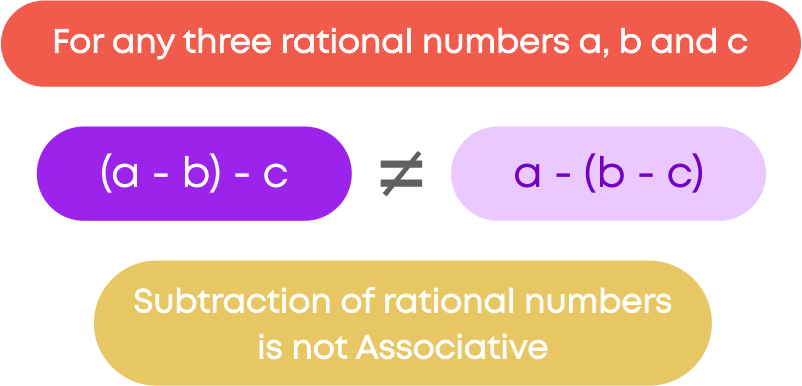Example: look at the expression given here

-5 9 – ( 4 9 - -2 11 )

Meena found that the denominators of the first two rational numbers are equal and for the ease of

calculation, changed the expression as ( -5 9 4 9 )- -2 11

Will she get the same answer as -5 9 – ( 4 9 - -2 11 )?

NO! In subtraction, if we change the grouping of the numbers, then the answer will change.

• When we multiply three rational numbers, ‘a’, ‘b’ and ‘c’, we can group the numbers to multiply.
• A change in the order of grouping does not change the product of the three numbers.
• Rational numbers are associative under multiplication.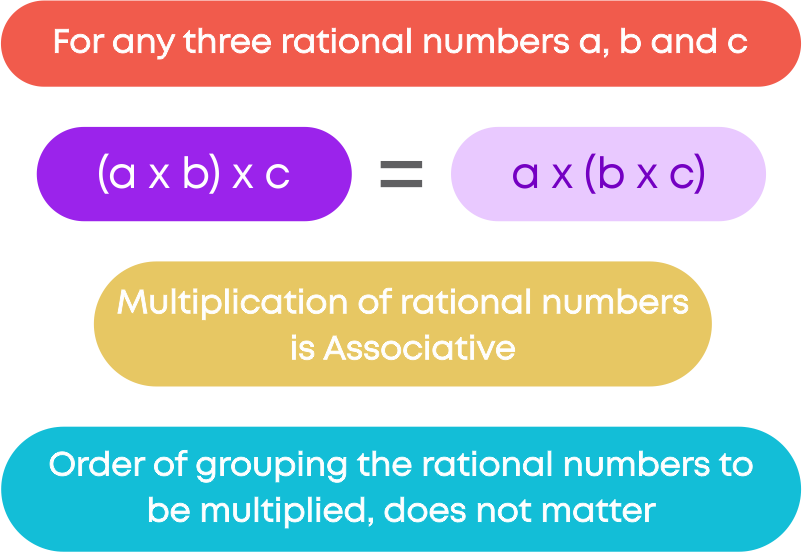We can use associative property to multiply rational numbers easily. First, identify the numbers which can be multiplied and simplified easily. Then, group these numbers using associative property. Multiply and find the result.

• When we are dividing three rational numbers, we can group the numbers.
• Changing the order of grouping will change the quotient.
• Rational numbers are not associative under division.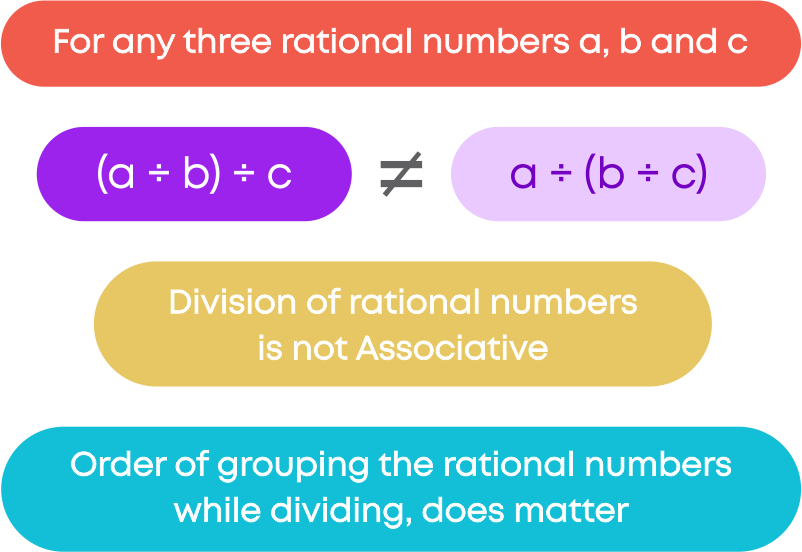The table below summarises the associative property for different number systems: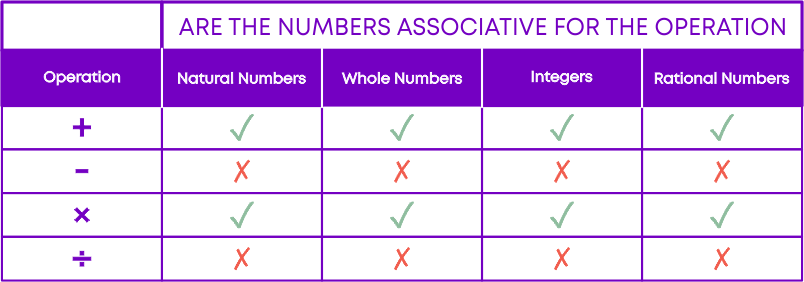#### Identity and Inverse

• When we add 0 to any number, the sum is the number itself.
• Zero is called the additive identity of rational numbers.

In general,

a + 0 = 0 + a = a, where ‘a’ is a rational number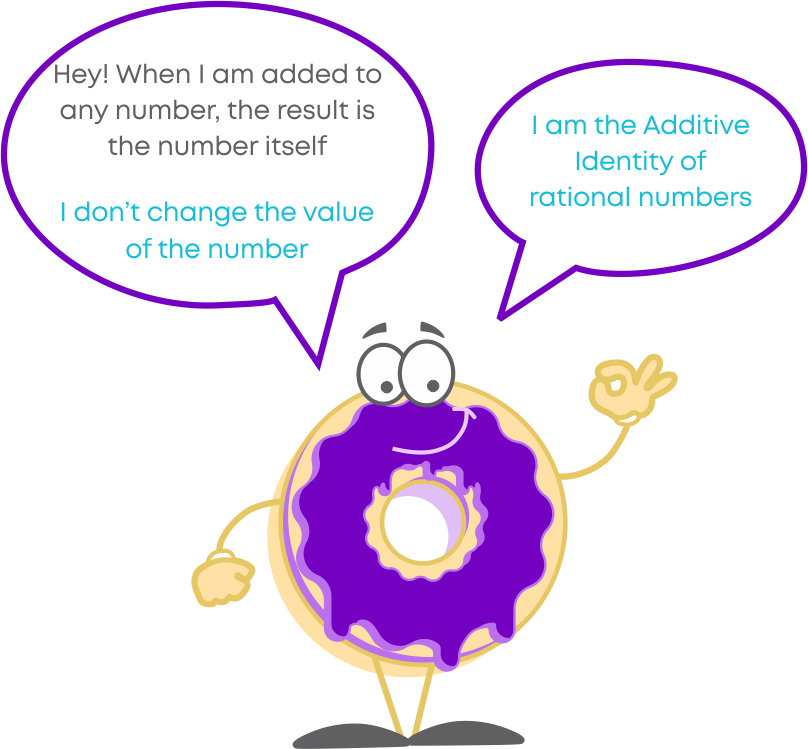When you add a whole number or an integer to 0, the result is the same whole number or integer itself. Hence, ‘0’ is the additive identity of the whole numbers and integers.

In general,

• a + 0 = 0 + a = a, where ‘a’ is a whole number.
• b + 0 = 0 + b = b, where ‘b’ is an integer.

Any integer or whole number multiplied by 1, results in the same number itself. This applies to rational numbers as well. For example:

-5 9 × 1 = -5 9 × 1 1 = -5 9

The product is the rational number itself.

Hence, 1 is called the multiplicative identity for whole numbers, integers, and rational numbers.

In general, a × 1 = 1 × a = a, where ‘a’ is a rational number.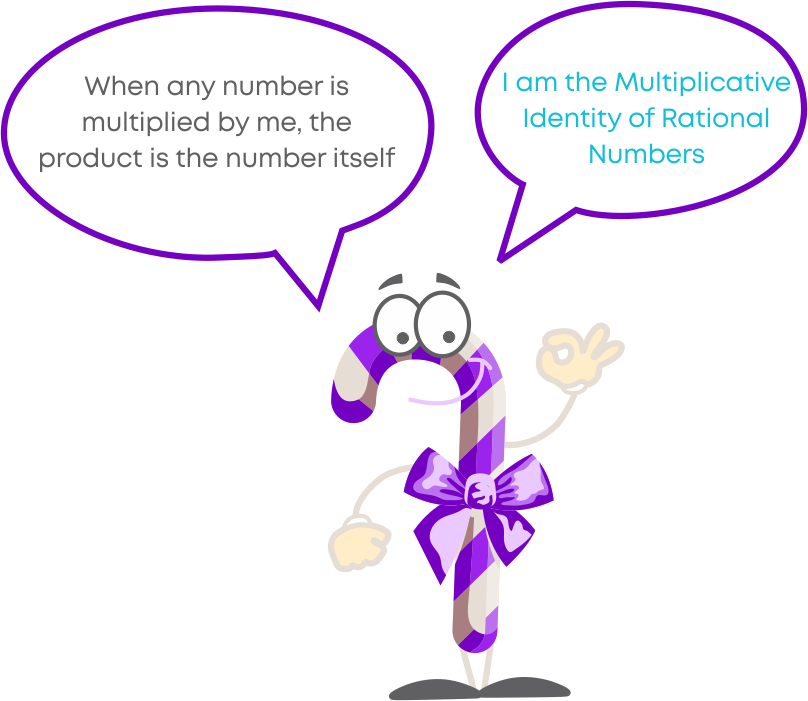Remember: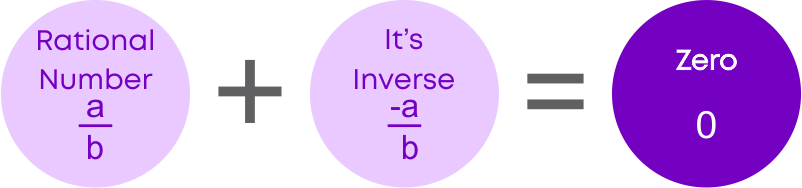From this, we can say that -a b is the additive inverse of a b . Similarly, a b is the additive inverse of -a b.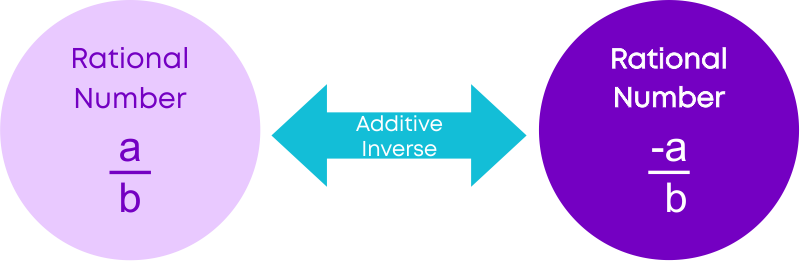The sum of a number and its negative is ‘0’. The negative is also called the additive inverse. The sum of an integer and its additive inverse always results in ‘0’.

The additive inverse of a rational number is its negative. The sum of a rational number and its negative is 0.

The multiplicative inverse of a rational number is its reciprocal. The product of a rational number and its multiplicative inverse is 1.

If a b is a rational number, then it must be multiplied by b a, so that their product is 1.

b a is called the reciprocal or multiplicative inverse of a b. a b is called the reciprocal or multiplicative inverse of ba.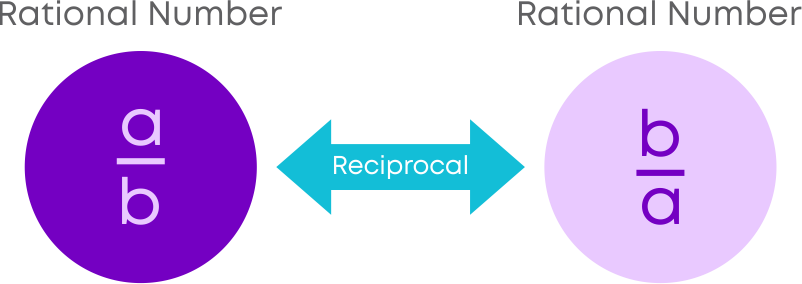The reciprocal of a rational number can be found by inverting the numerator and the denominator. It is also called its multiplicative inverse. Use the same rules of addition, subtraction, multiplication, and division while handling operations on a rational number and its reciprocal.

#### Distributive Property

When we must multiply a number with a sum of numbers, then multiplication distributes over addition. For three numbers a, b, and c:

a × (b + c) = (a × b) + (a × c)

Example: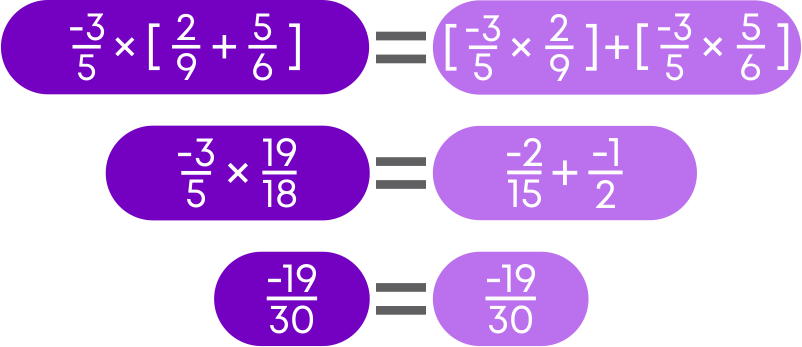Distributive property of multiplication over subtraction states that if ‘a’, ’b’ and ‘c’ are any three rational numbers, then

a × (b - c) = (a × b) - (a × c)

#### Representation of Rational Numbers on a Number Line

To identify fractions on a number line, we follow these steps.

• Find how many equal parts is the length between ‘0’ and ‘1’ divided into. This forms the denominator of the fraction.
• Identify the part where the fraction lies. This forms the numerator of the fraction.

To identify the rational numbers, we follow a similar strategy. For example, what is the rational number represented by the point X on the given number line ?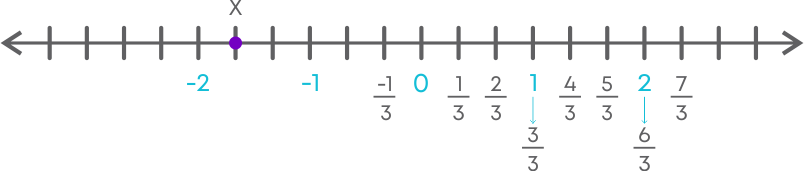Take a closer look at the number line. The distance between any two integers is divided into 3 smaller units. We know that each small unit is 1 3.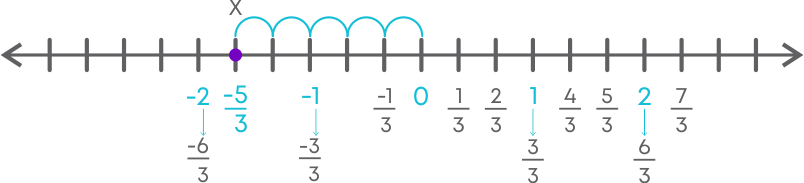The point ‘X’ is to the left of ‘0’ and hence negative.

Also, it is at a distance of 5 smaller units from ‘0’. This means ‘X’ represents the rational number -5 3.

To draw and represent a positive rational number on a number line:

• To represent positive rational number, move towards the right of ‘0.’
• The denominator of the rational number tells us the number of parts each unit on the number line should be divided into.
• Divide each unit into equal parts.
• Write the rational number represented by each division.
• Locate and mark the required rational number.

To draw and represent a negative rational number on a number line:

• To represent negative rational number, move towards the left of ‘0.’
• The denominator of the rational number tells us the number of parts each unit on the number line should be divided into.
• Divide each unit into equal parts.
• Write the rational number represented by each division.
• Locate and mark the required rational number

#### Rational Numbers Between Two Rational Numbers

You know that the mean of two numbers lies between the two numbers. Let us find the mean of the

two rational numbers, 2 5 and -1 3

• Add the rational numbers 2 5 and -1 3
• Divide the sum by 2.

2 5 + -1 3

= 6-5 15

= 1 15

1 15 ÷ 2

= 1 30

So, the mean of 2 5 and -1 3 is 1 30

Remember: if ‘x’ and ‘y’ are two rational numbers, then (x + y)÷2 is also a rational number between ‘x’ and ‘y,’ such that x < (x + y)÷2 < y.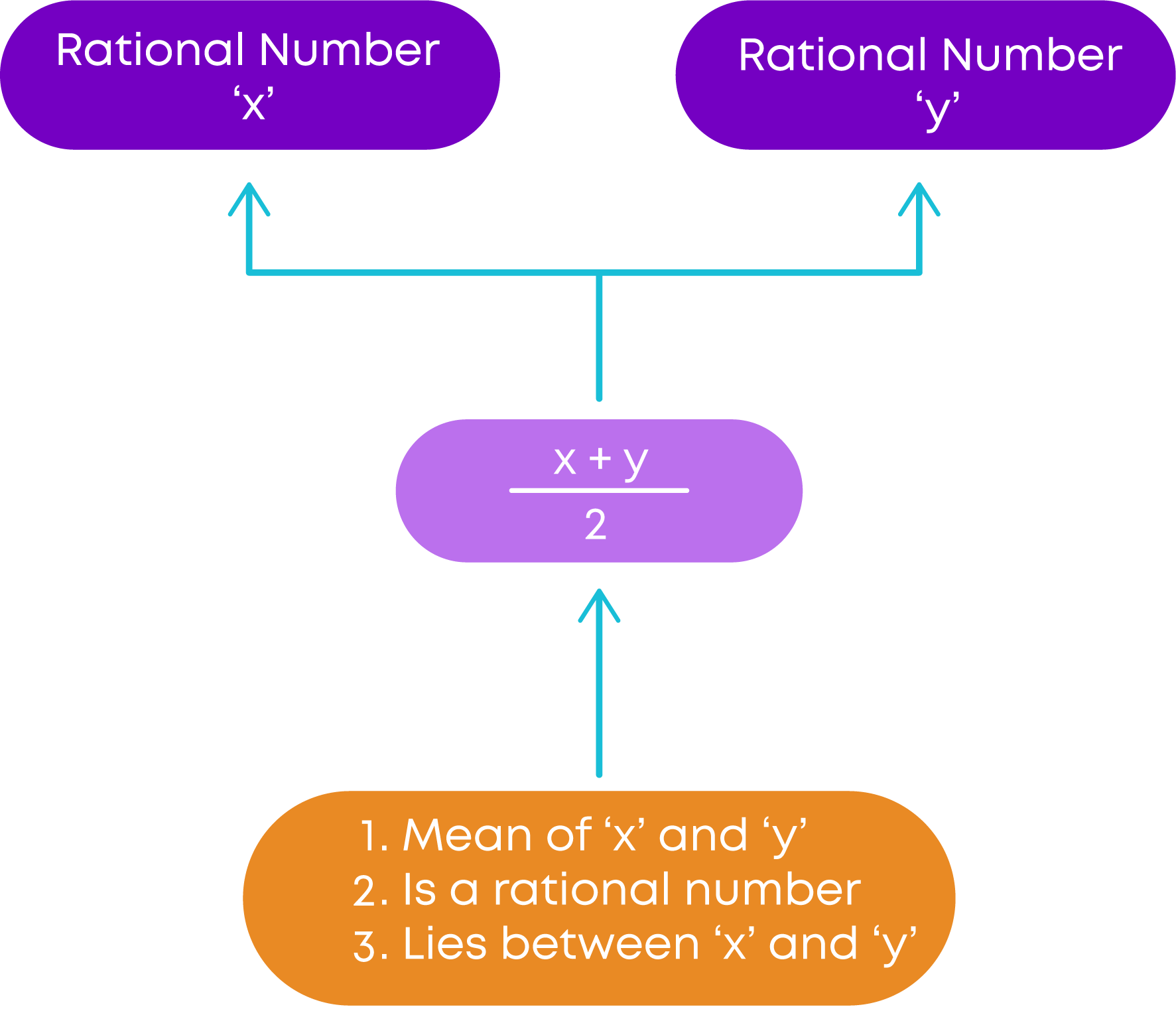To find a rational number between any two given rational numbers:

• Find the mean of the two rational numbers.
• Finding the mean of two consecutive rational numbers allows us to find infinite rational numbers between any two of them.

#### Common Errors

The following are topics in which students make common mistakes when dealing with rational numbers:

• 1. Operations with Rational Numbers
• 2. Apply Distributive property
• 3. Representing Rational Numbers on a number line

#### Operations With Rational Numbers

Operations with rational numbers will follow the rules of integers and fractions. When we simplify rational expressions, we must follow the rules of integers and fractions.

Example:

2 3 + ( -3 4 ) [ 8 9 × 6 11 ]

= 2 3 + ( -3 4 ) [ 16 13 ] → Follow rules of fractions to simplify the rational numbers

= 2 3 + -4 11

= 22-12 33 → Follow the rules of integers for performing the operations

= 10 33

#### Apply Distributive Property

To simplify expressions as given below, apply the distributive property.

a × b + c = a × b + a × c

a × b - c = a × b - a × c

Example:

-2 5 ( 5 12 + -4 3 )

Remember!

Multiply the term outside the brackets with each term within the brackets.

= -2 5 × 5 12 + -2 5 × -4 3

-1 6 + 8 15

= 11 30

#### Representing Rational Numbers On A Number Line

While representing a rational number like 1 5 , -3 8 , 2 7 , etc., remember:

• The denominator of the given rational number indicates how many parts should each unit on the number line be divided into.
• Draw a number line with each unit divided into equal parts as indicated by the denominator.
• Locate and mark the required rational number.

Example:

To locate -5 6 on the number line, we draw an integer number line. Here, the denominator is

6, hence, each unit on the number line should be divided into 6 equal parts.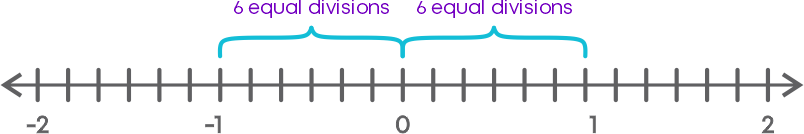Mark the required rational number.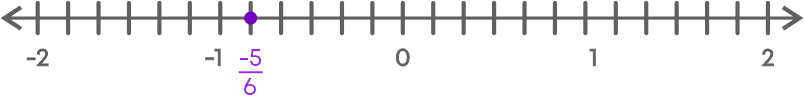#### Conclusion

Rational numbers are useful in our daily lives. Can you solve this riddle now that you have learnt about rational numbers?

Evaluate: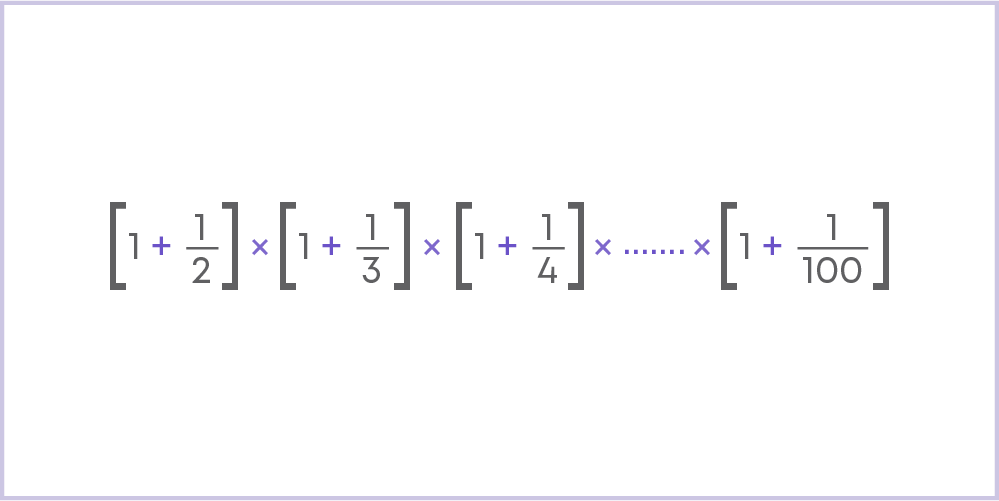#### Jyothi

Author

A math enthusiast and an educationist, Jyothi has a strong academic background and has demonstrated an impressive history of working in the education industry. Jyothi is skilled in developing and reviewing lesson plans, learning content, assessments, and video story boards and scripts.